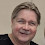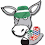## Tuesday, December 9, 2014

### NFL Picks Contest- Week 14 Results and Standings

Here are the Week 14 results and standings in the NFL Picks Contest. Captain Crunch and Grange gain more ground on the leaders.

Here are the current standings;

OneAndDone- 27+17+17+34+41+5+31+38+16+24+17+30+32+16 = 345 pts.
MOJO-  27+17+17+29+16 +24+32+37+16+34+17+30+32+16 = 344 pts.
cokeboy99- 27-(8)+21+34+16+13+20+38+16+51+43+13+32+16 = 332 pts.
Captain Crunch- 27+17+17+29+16+5+35+38+24+24-(17)+30+32+34 = 311 pts.
Grange95- (3)+17+21+29+16+24+11+20+16+0+43+13+62+34 = 303 pts.

Vegas Vic- 27+17+21+29+16+5+32-(3)+20+31+17+29+32+16 = 289 pts.
Music City Momma- 13+17+21+29+16-(7)+32+38-(3)+31+17+30+32+17 = 283 pts.
mrben09- 27+17+17+29+16+24+31+37-3+24-(15)+30+32+16 = 282 pts.
ggrouchie- 27+17+17+34+41+13+20-(3)+24+24-(15)+30+32+16 = 277 pts.
TwoMinuteWarning- (3)+17+37+29+16-(7)+35+37+20+24-(17)+19+32+17 = 256 pts.

lightning36- 27+17+17+29+16+24+31+24+20+34-(15)+30+32-(31) = 255 pts.
Neophyte- 27+17-(19)+0+41+24+21+0+24+24+17+14+32+24 = 246 pts.
Coach- 27+17+21+29+20+24+20-(3)+16-(7)-(20)+14+11+16 = 185 pts.
TeamMiRketti- 27+17+17-(3)+16+24+0-(19)+20+34+0+30+11+0 = 174 pts.

Week 14 scores

angerisagift- Arizona (W 17-14)= 13 pts.
MOJO- Green Bay (W 43-37)= 16 pts.
Vegas Vic- Green Bay (W 43-37)= 16 pts.
Captain Crunch- St. Louis (W 24-0) = 34 pts.
OneAndDone- Green Bay (W 43-37)= 16 pts.

mrben09- Green Bay (W 43-37)= 16 pts.
ggrouchie- Green Bay (W 43-37)= 16 pts.
Coach- Green Bay (W 43-37)= 16 pts.
TeamMiRketti-  0 pts.
lightning36- New Orleans (L 10-41) = (31) pts.

Neophyte- Houston (W 27-13) = 24 pts.
Music City Momma- Denver (W 24-17) = 17 pts.
TwoMinuteWarning- Denver (W 24-17) = 17 pts.
Grange95- St. Louis (W 24-0) = 34 pts.
cokeboy99- Green Bay (W 43-37)= 16 pts.

Thanks for playing.

Week 15 picks are due by Saturday at 6pm (CDT). Only Sunday and Monday games are eligible.

Good luck!

1.2.